# Do The Math Q21

The future lifetimes (in months) of two components of a machine have the following joint density function:

What is the probability that both components are still functioning 20 months from now?

1.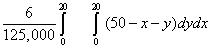2.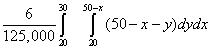Correct Answer
3.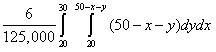4.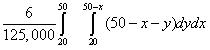5.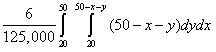Solution: B

We are given that: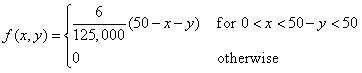and we want to determine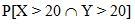.

In order to determine integration limits, consider the following diagram:

We conclude that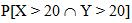=